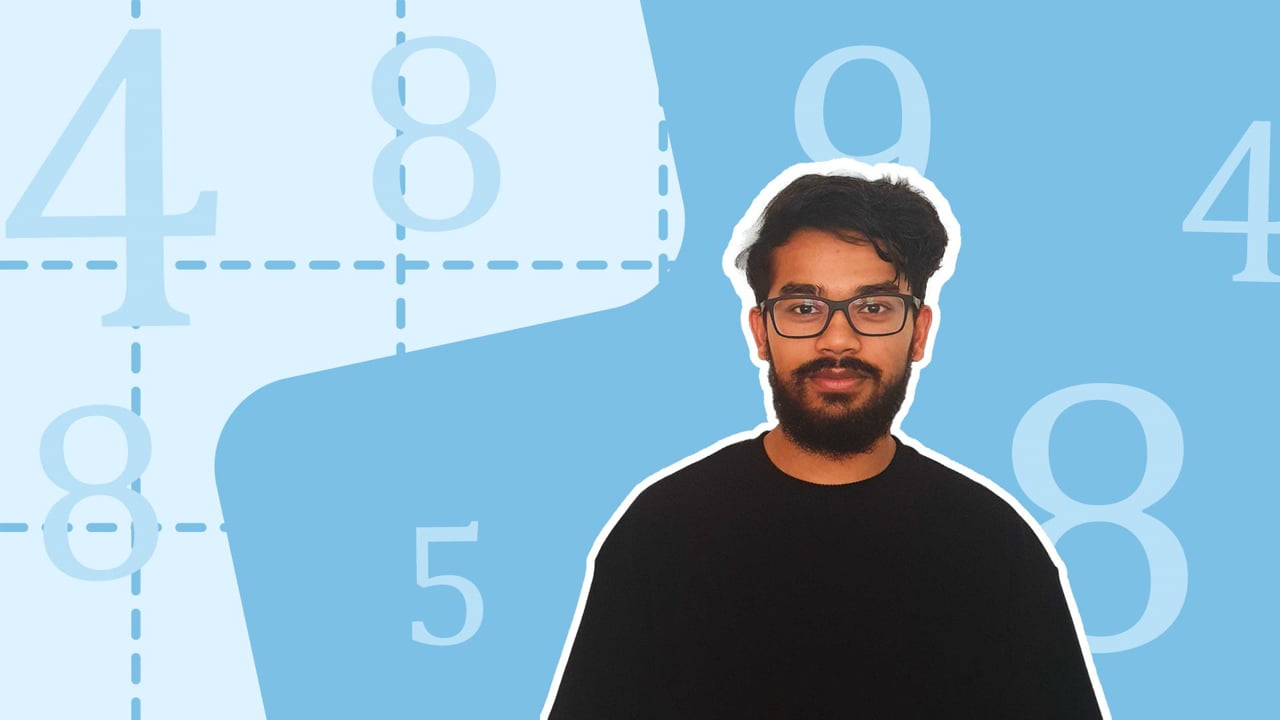Chapter overviewMaths

Types of numbers

Number calculations

Fractions, decimals and percentages

Algebraic manipulation

Formulae and equations

Straight line graphs

Other graphs

Ratio

Proportion

Rates of change

Shapes

Properties of shapes

Measures

Lines and angles

Drawing shapes

Trigonometry

Probability

Statistics

Maths

# Percentage change0%

Summary

# Percentage change

## In a nutshell

Percentage change compares how much things have either grown or deteriorated. It can be used to calculate values of objects before and after they undergo a percentage change. However, it is best to first learn about multipliers.

## Multipliers

A multiplier measures how much a quantity has changed by comparing the new value to the old one. A multiplier greater than $1$​ suggests an increase in value, whereas a multiplier less than $1$​ suggests a decrease in value.

### Formula to calculate the multiplier

The multiplier follows the formula below:

$\text{new\,value}=\text{multiplier}\times \text{initial\,value}$

##### Example 1

The value of a car has increased from $£2000$​ to $£2500$​. What is the multiplier of this change?

Substitute values into the equation:

\begin{aligned}\text{new\,value}&=\text{multiplier}\times \text{initial\,value}\\2500&=\text{multiplier}\times 2000\end{aligned}​​​​

Rearrange and solve for the multiplier:

\begin{aligned}\text{multiplier}&=2500\div2000\\&=1.25\end{aligned}​​

The multiplier is $\underline{1.25.}$

### Calculating the multiplier using percentage change

You may be given the percentage increase or decrease in a question and will need to calculate the multiplier.  In this instance, use the following steps:

#### Procedure

 1 Convert the percentage into a decimal by dividing it by $100$​. 2 Establish whether you have a percentage increase or decrease. 3 If you have a percentage increase, add the value from Step One to the number $1$​​ If you have a percentage decrease, subtract the value from Step One from the number $1$​.

##### Example 2

The cost of a certain brand of chocolate has increased by $10\%$. Its new price is $£2.20$. What was its original price?

Firstly, calculate the multiplier.

\begin{aligned}10\%&=0.1\\\text{multiplier}&=1+0.1\\\text{multiplier}&=1.1\end{aligned}​​

Substitute the multiplier and the value in the question into the equation below:

\begin{aligned} \text{new\,value}&=\text{multiplier}\times \text{initial\,value}\\£2.20&=1.1\times \text{initial value}\\\dfrac{£2.20}{1.1}&=\text{initial value}\\\text{initial value}&=£2.00\end{aligned}​​

The original price was $\underline{£2.00}$.

## Percentage change

To find the percentage change, you are calculating by what percentage of the original value something has increased or decreased.

### Percentage change formula

To calculate percentage change, use the following formula:

$\text{percentage \ change} = \dfrac{\text{new \ value} - \text{initial \ value}}{\text{initial \ value}} \times 100$​​

Note: A positive value for percentage change indicates a percentage increase, while a negative value indicates a percentage decrease.

## Percentage change problems

You may be asked to find the value of a quantity after it changed, before it changed, or be asked to find the percentage change itself. To do this, use the percentage change formula.

##### Example 3

Identify and calculate the percentage change from $200$​ to $130$​.

Substitute the numbers into the formula

\begin{aligned}\text{percentage \ change} &= \dfrac{130-200}{200}\times100\\&= -\dfrac{70}{200}\times100\\&=-35\end{aligned}

Note the sign in front of the value to establish whether the change is an increase or decrease.

It is a percentage decrease of $\underline{35\%.}$

## Simple interest

Simple interest is an application of percentage increase. It is about increasing the value of something by a fixed amount at regular intervals (usually once a year). It is best understood with an example.

##### Example 4

The value of a car increases with simple interest of $10\%$​ a year. If the car is initially worth $£5000$​, how much is it worth after $8$​ years?

Work out the monetary increase for one year:

$10\%$​ of $£5000$ is $0.1\times£5000=£500$

The car increases in value by $£500$ per year.

Work out the increase for $8$​ years:

$1$​ year = $£500$ increase

$8$​ years = $£500 \times8 =£4000$ increase

The car increases in value by $£4000$ after $8$​ years.

Work out the total value after $8$​ years:

$£5000+£4000=£9000$​​​

The car is worth $\underline{£9000}$ after $8$​ years.

FAQs

• Question: What is simple interest?

Answer: Simple interest is increasing the value of an object by a constant amount in regular intervals.

• Question: How do you calculate a multiplier using percentage change values?

Answer: Firstly, convert the percentage to a decimal by dividing it by 100. Then, if you have a percentage increase, add this value to the number 1. If you have a percentage decrease, subtract this value from the number 1.

• Question: How do you calculate the percentage change?

Answer: Percentage change = (new value - initial value)/initial value * 100

Theory

Exercises